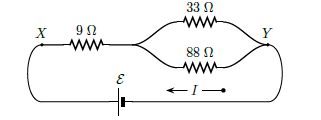# Problem: The following diagram shows part of a closed electrical circuit. Find the electric resistance RXY of the part of the circuit shown between point X and Y. 1. RXY = 33 Ω 2. RXY = 35 Ω 3. RXY = 45 Ω 4. RXY = 25 Ω 5. RXY = 21 Ω 6. RXY = 31 Ω 7. RXY = 43 Ω 8. RXY = 23 Ω 9. RXY = 29 Ω 10. RXY = 7 Ω

🤓 Based on our data, we think this question is relevant for Professor Ponting's class at North Carolina A & T State University.

###### Problem Details

The following diagram shows part of a closed electrical circuit. Find the electric resistance RXY of the part of the circuit shown between point X and Y.

1. RXY = 33 Ω

2. RXY = 35 Ω

3. RXY = 45 Ω

4. RXY = 25 Ω

5. RXY = 21 Ω

6. RXY = 31 Ω

7. RXY = 43 Ω

8. RXY = 23 Ω

9. RXY = 29 Ω

10. RXY = 7 Ω